## Mathematical Vindication!

Some time ago I blogged here about the absurdity of the infinite sum 1+2+3+4… = -1/12. I did a partial analysis here but I never really completed my thoughts. I’m lazy.

Anyway I noticed today a great explanation by Mathologer on youtube. I wont explain here his logic but it closely matches what I was thinking at the time. Go see his video. Basically the idea that the infinite series 1-1+1-1+1-1… is a number at all is simply wrong. So using it to determine the sum 1+2+3+4… is wrong. As I said at the time, maths getting derailed.

What is curious is how we can use numbers and operations on numbers to construct something that is not a number. There is no problem with this. Most agree that 1/0 is not a number. Some would argue the ‘number’ is infinity. OK.. but does 1/0 + 1/0 = 2 x infinity? No. Regular numeric operators (+, -, *, /) don’t apply. So if you sum s = 1-1+1-1+1… doesn’t equal a number, you can’t expect adding and subtracting other infinite series from it makes sense.

’nuff said.

## Random Number Generation Part II – Some Data Analysis

Following on from my last post, I’ve been trying to read the voltage values that are generated from the random number generator I put together. I was initially using an arduino unit so I could control the voltage output down to below 1 volt. Ultimately I want to read the values with an ESP8266 unit running micropython so I can upload the values to an internet based data logger and the ESP8266 is only 1 volt tolerant on its analog-to-digital pin. So, the voltage has to be controlled and I didn’t mind blowing up one of my arduinos (which are 5 volt tolerant). The voltage divider is now composed of a 10k resistor from positive rail to analog out and a 1k resistor from analog out to ground.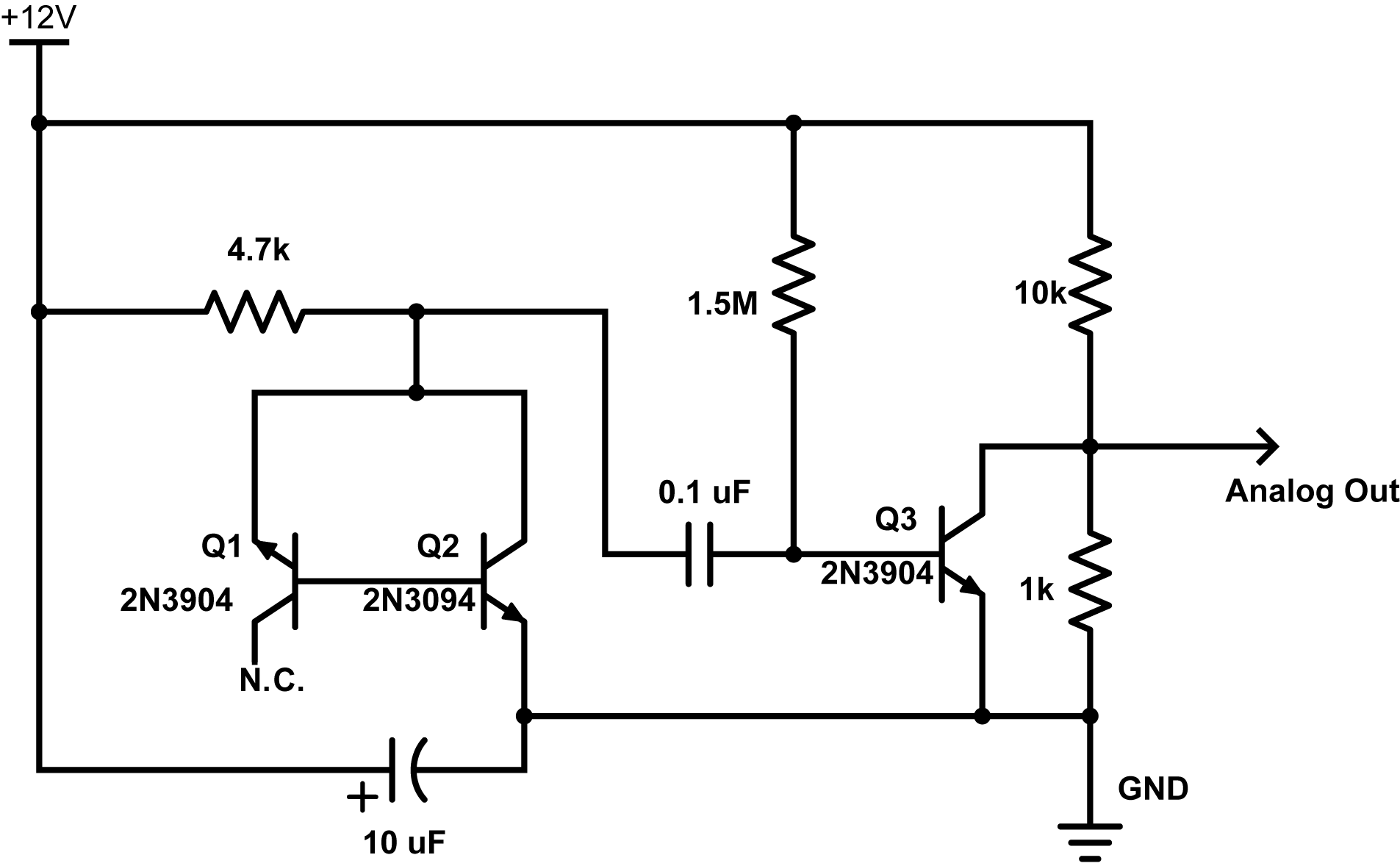The ardunio was giving me readings up to about 180 unit out of 1024 which gives about 180/1024 * 5V = 0.8789 volts… nice and safe.

Reading these voltages with the ESP8266 gave some interesting results. The code to do this was very simple:

from machine import ADC

import time

for i in range(1000000):
time.sleep_ms(10)

This reads a voltage every 10 milliseconds from the analog pin and reports one million of them. It took about 2.8 hours and the output was redirected to a file of type csv with this command on my mac:

ampy --port /dev/tty.SLAB_USBtoUART run readanalog.py > avalanche_noise.csv

I then analyzed this data in python using the jupyter web interface. A histogram plot of the values looks like this: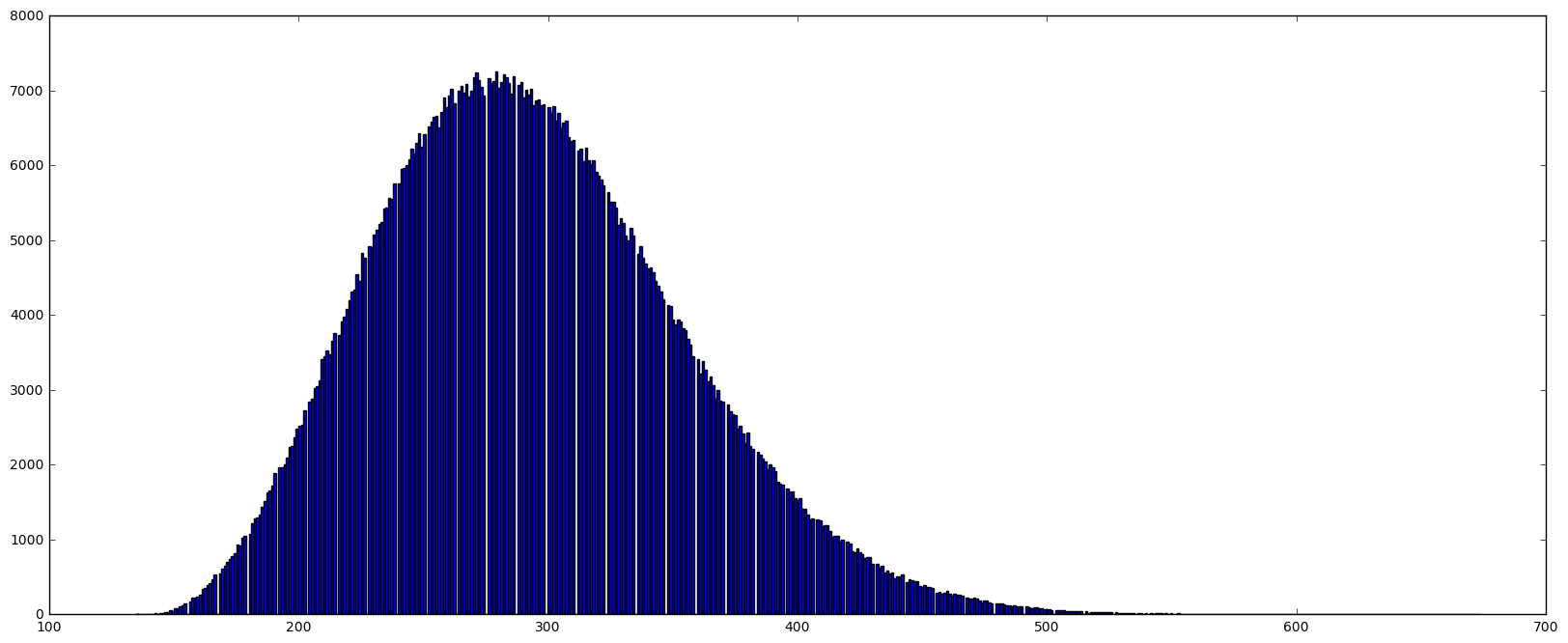The values range from 135 to 674 with a mean of ~292.6. This distribution is obviously skewed too so it’s not gaussian distributed – maybe Poisson?  But the most striking thing is the lines of empty space (no blue) – is this a plotting problem or are certain values skipped during the reads? Well lets zoom in around the top of the distribution.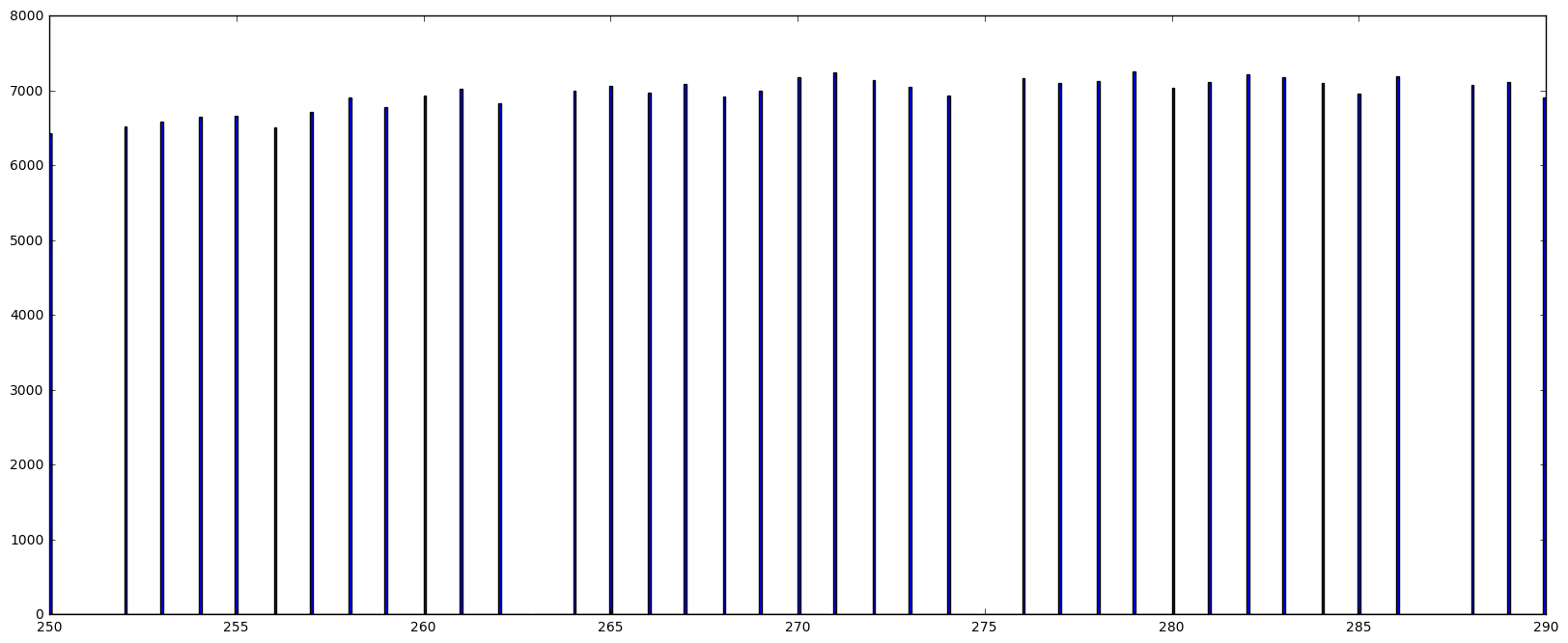So its true, some values are just never recorded. Its hard to believe the voltages from the circuit would do this. Also, its clear from the numbers that every 12th value is skipped. I think this must be an error in the way micropython reads the register so I’ll probably post this somewhere in the micropython forums and see if this is true or if I’m just doing something wrong.

Next I tried to fit this distribution to a gaussian curve. It failed. Here is the python code:

import math
import numpy as np
import matplotlib.pyplot as plt
import scipy as scipy
import scipy.stats as stats
from scipy.optimize import curve_fit
from scipy.misc import factorial
from scipy.stats import norm
%matplotlib inline          # needed for plotting in jupyter

data_p = data # not really needed

fig = plt.figure(figsize=(20, 8))
entries, bin_edges, patches = plt.hist(data_p, bins=(int(np.amax(data_p)-np.amin(data_p))), range=[np.amin(data_p),np.amax(data_p)], normed=True)
bin_middles = 0.5*(bin_edges[1:] + bin_edges[:-1])

# poisson function, parameter lamb is the fit parameter
def gauss(x, *p):
A, mu, sigma = p
return A*np.exp(-(x-mu)**2/(2.*sigma**2))

# fit with curve_fit
p0 = [1., 260., 30.] # initial guesses for height, mean and SD

parameters, cov_matrix = curve_fit(gauss, bin_middles, entries, p0 = p0)
print parameters # print results
print np.sqrt(np.diag(cov_matrix)) # print errors of fit

# plot poisson-deviation with fitted parameter
x_plot = np.linspace(0, np.amax(data_p), 1000)
plt.plot(x_plot, gauss(x_plot, *parameters), 'r-', lw=4)
plt.show()

Output: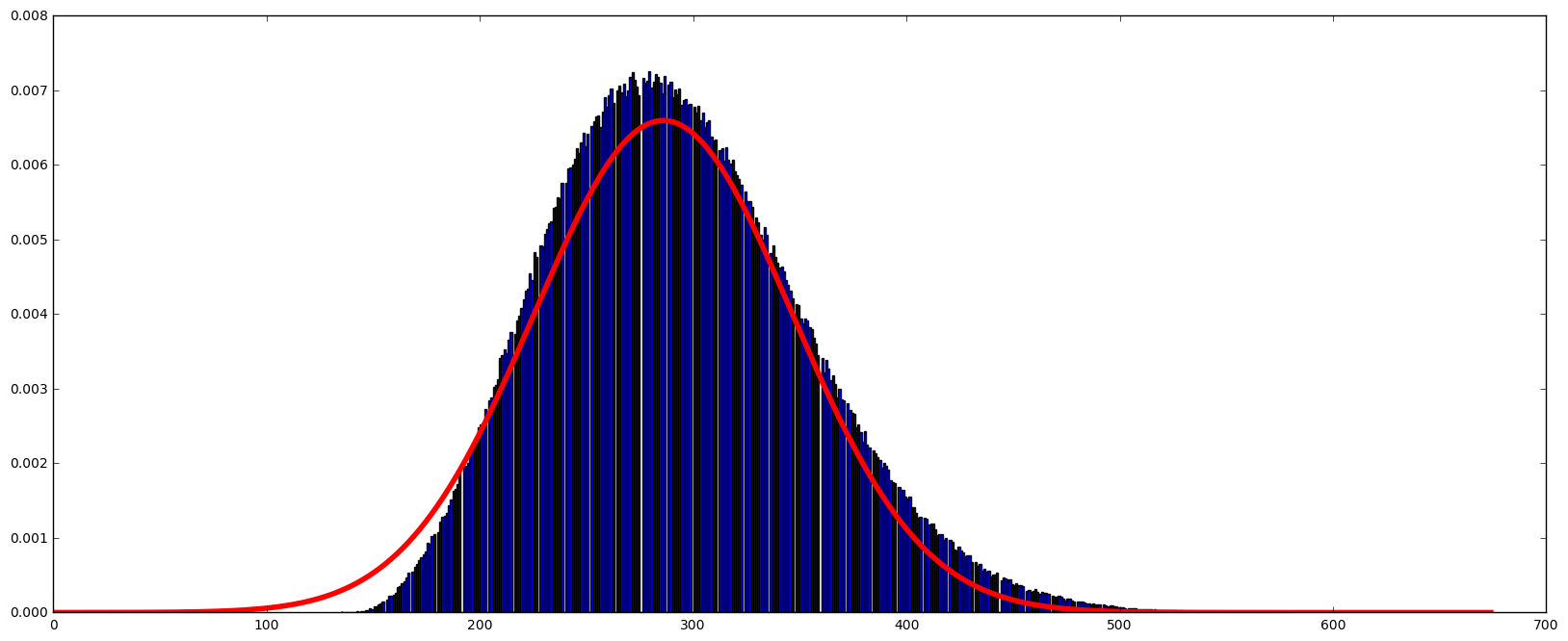This doesn’t fit very well. But it does reveal the skew present in the data. It does in some ways look poisson distributed (you can read more about this distribution here) and electrical noise is typically poisson distributed because of the discrete nature of electrical charge (read about this and shot noise here).

Mathematical aside:

One way I like to think of the Poisson distribution and Poisson processes is as follows. They arise from discrete events that must always have a positive value – this is not true for Gaussian distributions. So, in our circuit, current (electrons) flow or do not flow. When they flow, a discrete (integer) number of them flow. There is a lower limit on the number that can jump the gap. That number is zero. The gaussian distribution is the limit of a binomial distribution as the number of events goes to infinity. The binomial distribution is based on the idea that an event either happens or does not. (N.B. this is different from the Poisson case because in that case, events either happen 1 or more times or do not happen). If we accumulate binomial events, there is no limit to how many ‘yes’ or ‘no’ events can happen so some of the distribution must extend as far as the number of events recorded – for gaussian this limit goes to infinity and negative infinity. The Poisson distribution doesn’t act this way. If we try and model the Poisson distribution as an infinite binomial distribution we quickly realize that while we can get an infinite number of ‘zero value’ events as well (with low probability) there is more than one other alternative. So the distribution must take into account these many possible values which stretches the distribution in the positive direction while there is a still a hard limit at zero. We can shift the Poisson distribution so ‘N’ zero-value trials will be plotted at ‘-N’ (like we would for a binomial distribution) but on the positive size, the curve would extend past ‘+N’ because some of those trials can have a value of more than 1.

Enough qualitative description of distributions…

Using a poisson function instead like this:

def poisson(k, lamb):
return (lamb**k/factorial(k)) * np.exp(-lamb)

and we get this: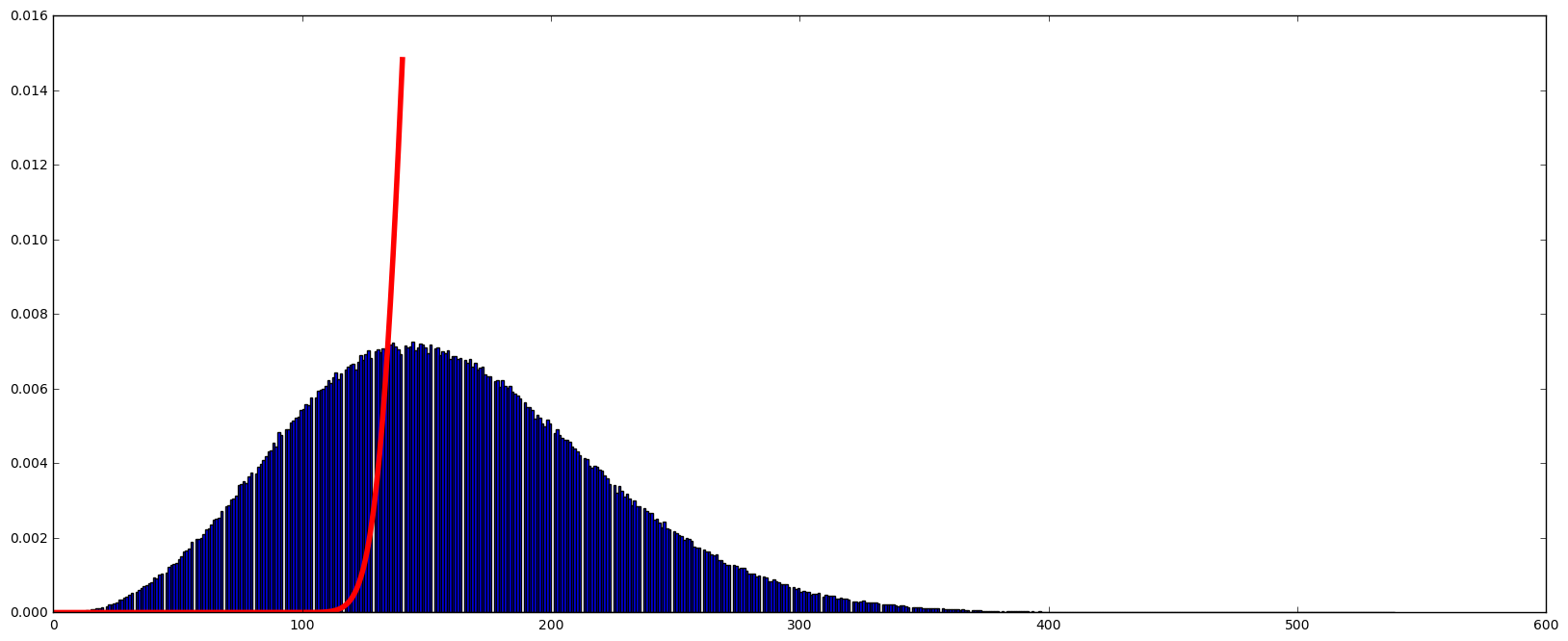It doesn’t converge at all.

Yikes, whats going on?

Well my thought was that the numbers I’m reading don’t match the number of electrons actually flowing. This signal is amplified by the transistors and then quantized, not in nature, but by the ADC converter in the ESP8266. My hope is that this signal is proportional to the number of electrons that flowed during signal acquisition. But this signal is not the same as the number of electrons which will be behaving as a Poisson process. But the recorded numbers should be proportional to the number of electrons. So if we divide these values by some constant, can we get the fit to work, and at what optimal division factor.

Long story short, if I divide the 1000000 million points by 23.9 I get an optimal fit in terms of the error reported for the fitted parameter. That parameter, which is the mean value, is ~6.558336. Does this mean, that on average 6.5 electrons pass through the transistor while the ESP8266 is taking an ADC measurement? I think it might be! Here is the fit: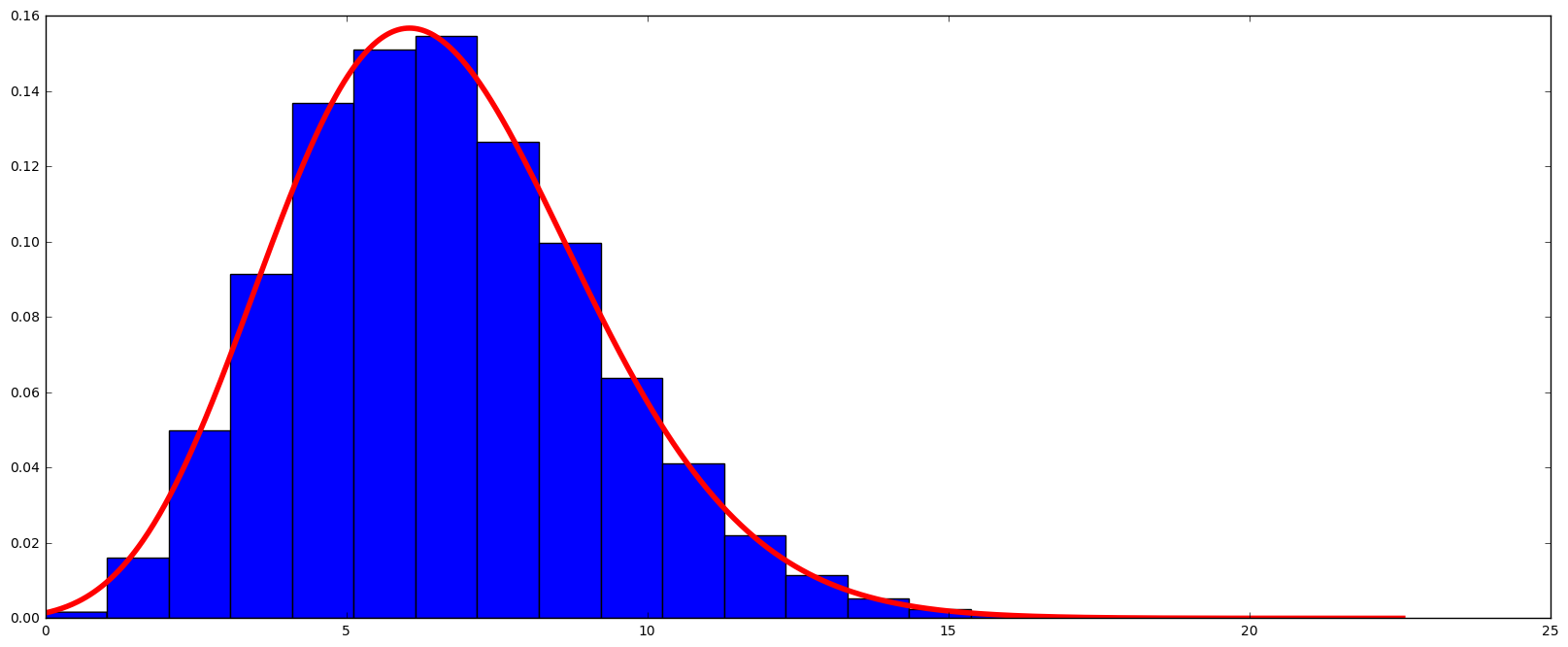If I take these same numbers and try and fit a Gaussian curve, it doesn’t do as well.Conclusions? The electrons that flow across the junction in reverse bias are behaving as a Poisson process as expected. The distribution is not flat. I’ve seen some discussion on the net where people seem to assume this would be the case. It does seem to be random! One of the next steps is to convert these numbers to a flat distribution or at least make it generate a binary sequence. It seems to be that XORing  or Von Neumann filtering will not do a good job of removing the biasing that the Poisson distribution will introduce.

## Lotteries and “Getting what you are looking for”

I came across a review/excerpt in Scientific American for a new book called “The Improbability Principle: Why Coincidences, Miracles, and Rare Events Happen Every Day”. This article has an excellent description of how seemingly impossible events can and do take place all the time.

The major point of the excerpt was that some events like finding people with matching birthdays can be a rare event or a common event depending on what you mean by a matching event. In a room of 23 people there is about a 6% chance one of them will share a birthday with you, but a greater than 50% chance that two of the 23 people in the room will share a birthday (see a description of the birthday problem here). An intuitive explanation for this is when you’re looking for someone who shares your birthday you have limited the possibilities – they must match your birthday – but when we allow anyone to match any other person, the possible number of combinations of people matching birthdays goes up a lot. So its a lot more likely. The maths for this is at the above link. I don’t need to repeat it here. The point is, a subtle change in the question can have big consequences for the answer.

The excerpt then goes on to talk about the Bulgarian Lottery coincident that occurred in 2009. In one draw, the winning numbers were 4, 15, 23, 24, 35, 42. “Amazingly” the next draw, the same numbers again. There was outrage with people calling the draw rigged and there was even a full government investigation. As an aside, if you could rig the draw why would you repeat the numbers? Seems silly to do that. Why not just rig the draw with some other numbers? Anyway…. the question is, how surprised should we be that this happens at all?

The Bulgarian lottery is a 6 number draw from 49 numbers. So the probability of getting the right numbers is 1/(49!/(6!*43!)) or one in 13,983,816. Once you have a particular set of numbers the chance that any other specific draw will have the same numbers is one in 13,983,816 – its the same as picking the numbers in the first place. This seems like a pretty unlikely event. But this is like finding someone who has the same birthday as you. You are trying to match one specific draw to another. What happens when you have many draws and you are trying to find a match between any of those two draws? The Bulgarian lottery has been going on for a while, so there have been many draws and so the chances that a match would happen among all these draws is much less amazing. For example, among 50 draws there are 1,225 ways we could match 2 of the draws. Quickly, the number of ways to find a match goes up and as a result the probability of a match happening goes up as well.

Now some skeptics might say, “But this was two draws in a row!!! Not a draw this week matching a draw from 5 years ago. This is very special because of the serial way in which it happened!”. I felt this objection was glossed over in the SciAm excerpt. Thinking that the consecutive nature of the draws is special in this case is thinking about it all wrong. Actually its thinking yourself into the special circumstance. You are saying that the two draws in a row is a special thing. But this is the same as finding that one person who matches your birthday.

Another way to think about this sort of thing is to consider the following. Instead of drawing 4, 15, 23, 24, 35 and 42, imagine the lottery drew 1, 2, 3, 4, 5 and 6. Would you think this was amazing? Rigged? Would it be all over the internet and in all the papers? Yes it would. But is it any more unlikely than 4, 15, 23, 24, 35 and 42? No, its not. They have the same chance of happening… one in 13,983,816. The only reason it would be considered special is because the numbers are consecutive. By why is that any more special than starting at 4, adding 11, adding 8, adding 1, adding 11 again and then adding 7? Its only because of the value placed on consecutive numbers by our minds. We humans would say consecutive draws has more meaning or is more special than one draw matching a draw from 5, 10 or 15 years ago. In terms of random events neither is more special than the other.

Your perspective influences your expectation, but not reality. Uncommon things will still happen all the time, just not the sort of uncommon things you might expect to happen.

## When Maths Gets Weird – and Maybe Derailed$\sum_{i=1}^{\infty}i = 1+2+3+4...$ obviously doesn’t converge. Right? This sum doesn’t actually evaluate to a number, does it? Well I wouldn’t think so – no part of my intuition suggests this series is limited. So imagine how surprised I was (and perhaps you too) when I learned this Maths conundrum has been spreading around the internet early this year due to a few videos from the Numberphile guys. Their answer?$\sum\limits_{i=1}^{\infty}i = 1+2+3+4... = -\dfrac{1}{12}$
What? Really?$-\frac{1}{12}$? There is something odd here, clearly. How can this be true? This is even beyond breaking intuition, this is just plain wrong. So why is this even taken seriously? The ‘proof’ comes from a field of Maths called Mathematical Analysis and infinite series. I wont go over the proof, there are examples here, here and here. However,  they all depend on the following equality:$1-1+1-1+1-1.... = \dfrac{1}{2}$

This is true for a Cesàro summation. That is, its true because of a definition of how to sum a series that doesn’t actually converge to a value. This is fairly well accepted and I suppose if you define the process of Cesàro summation to be a method of summing non-converging sequences then that is just fine… by definition. For me, however, this doesn’t sit right. This is not a sum that comes from the axioms that lead to arithmetic, the so called Peano axioms (I don’t have problems with the Peano axioms – I doubt you do/would too). But I do have a problem with Cesàro summation as a definition. Since Cesàro summations have results like 1-1+1-1… = 0.5 that contradict other forms of the sum like…$(1-1) + (1-1) + (1-1)... = 0 + 0 + 0... = 0$

and$1 - (1+1) - (1+1) - (1+ 1)... =1 - 0 - 0 - 0... = 1$

the only way the sum could be evaluated to 0.5 is by a specific definition that excludes the above sums. But why should I select this definition? Do I accept it as an axiom? If I do, how do I account for the fact that this axiom has two other intuitive sums? Obviously it is a bad axiom and therefore a bad definition. I really don’t understand why anyone would accept the Cesàro summation as anything useful at all. It immediately leads to inconsistencies. Its useless.

More to come….

## NMR, Compressed Sensing and sampling randomness Part II

##### Using Compressed Sensing: Selecting which points to collect.

NMR data is collected as a vector or matrix of points (depending how many dimensions the experiment has). Compressed sensing permits us to collect a subset of the actual points that are usually required. The question is, how do you decide which points to collect.

TL;DR Summary: Compressed sensing (CS) allows us to sample a subset of the points normally required for an NMR spectrum. CS theory suggests we should sample these points randomly. Random sampling leads to problems with the distribution of the gaps between samples. There is a better solution for NMR data.

##### The problem of Random Sampling.

Formal Compressed Sensing (CS) theory says we should collect a random spread of points between 1 and the final point (N). We could make a schedule of points by constructing a “dart-throwing” algorithm that randomly selects a point between 1 and N. If the algorithm selects a point twice, we just ask it to select another time. We run this until we have N/5 points. Surprisingly, it turns out this does not work well. Lets see why…

Below is an example of what happens when you select randomly 64 points out of 256 points. First of all, to make sure I selected points in an unbiased way, I took the numbers 1 through 256 into a vector and did a Fisher-Yates shuffle. Then I simply select the first 64 points in the vector after the shuffle. It has been shown that the Fisher-Yates shuffle is unbiased and I think it is a better solution to sampling purely randomly than a dart-throwing algorithm. Since I have 64 random points out of 256, you would expect that the average distance between numbers should be:

(256 – 64)/65 = 2.95385

This is because we need to eliminate 64 out of 256 (256 – 64) because they are selected and not part of gaps, then we must divide by 65 because by selecting 64 points we actually create 65 gaps. This means that the average gap size (that is the gap between selected numbers) is 2.95385.

I did 45 simulations like this, generating 64 * 45 = 2880 gaps. The average gap was 2.96319 so this basically looks good. Now, the Standard Deviation was 3.35334 which shows something funky is happening right away. This is a very large standard deviation for a mean value of 2.95385 and permits negative numbers to appear within one standard deviation. This can’t even happen for a gap as  gaps can’t be negative and there are no negative gap values in my numbers. How can this standard deviation be? Clearly the gaps are not normally distributed. Lets look at a histogram of the gap sizes.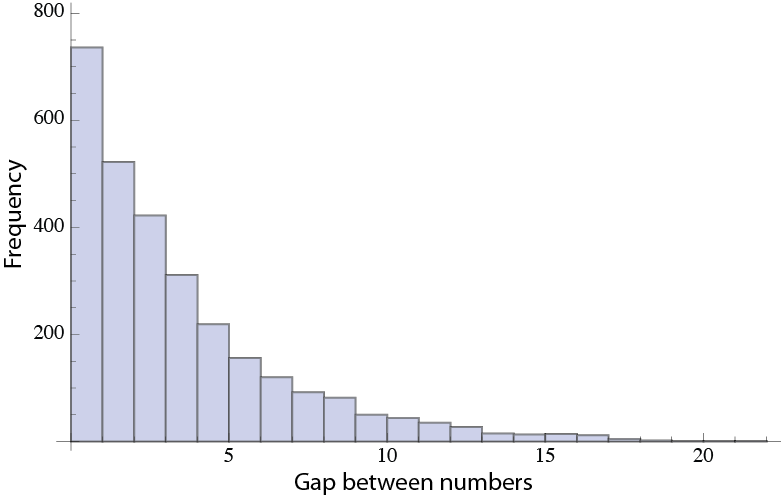Yikes, its an exponential distribution. This has been noted before for random gap sizes, but I was amazed when I first saw this – in hind sight I’m not, but at first I was a little shocked. To confirm that this really does happen for random sampling I went looking for another example of a subset of random numbers selected from a pool of numbers. Lottery results of course are a good example.

I acquired the complete set of Megamillions lottery numbers from about June 2005 to October 2013 (the nature of the pool of numbers did not change in that time) and calculated the gaps between these random numbers. In this game you had to pick 5 numbers from 1-56 (the rules have since changed). So the average distance between numbers is

(56 -5) / 6 = 8.5

My calculated gap distance is 8.42009 and the histogram of the gap sizes is below.So, why does this matter? Well what is happening is the gaps are distributed in a way which can cause problems when we try and fill in the data we skipped in the gaps. To begin with look at the above histograms. When we randomly select points we end up making gaps such that the most common gap size is actually no gap at all. Thats pretty remarkable when you think about it. Then the second most common gap is the smallest gap, a gap of 1. By the time we have reach the average gap (around 8 for Megamillions, or a little less than 3 for selecting 64 points from 256) one half of our gaps have been accounted for, by definition. The reason so many gaps fall here is because there is a lower limit to the size of the gap. They can’t be less than zero. Now, all these small gaps are great, because they should be easy to fill in. The problem is the upper limit on the gap size is the size of the sampling space minus the number of samples. This number is always going to be several times larger than the average gap size. Simply put, this will always give the exponential distribution seen in the histograms, resulting in some gaps that are much larger than the average gap size. These large gaps present problems when trying to fill in data with predictive methods.

So how do we do better than this when ‘randomly’ selecting which samples to take? First of all, we don’t use a straight random algorithm – it makes gaps that are too large. Instead we introduced some randomness around the average gap size by using a poisson distribution. We call this Poisson Gap sampling, a method developed by Sven Hyberts in my lab.

Details will be in the next post.

## NMR, Compressed Sensing and sampling randomness Part I

One of the things I spend my time thinking about is the problem of shortening the time it takes to acquire Nuclear Magnetic Resonance data on biomolecular macromolecules like proteins and nucleic acids. Below is an attempt to describe the problem non-technically and how we can speed up data acquisition by ‘randomly’ sampling a subset of the data normally required.

TL;DR Summary: Acquiring nuclear magnetic resonance data takes a long time. This is because to get good resolution traditionally a certain number of data points must be sampled.  Can we skip some of these points? The answer is yes, the next question is which points?

##### The problem: NMR takes a long time.

NMR spectra have great power in describing macromolecules at the atomic level when collected in 2, 3 or even 4 dimensions. Each dimension represents a different kind of information (say, nucleus type, location in a repeating unit of the polymer, distance from another nucleus) – so multiple-dimension spectra are data-heavy but help isolate specific atomic groups really well. The information in each dimension is actually frequency information – it is the frequency of each atom in the molecule. The down-side is these spectra take a long time to acquire. In fact, to acquire 3 and 4 dimensional data, experiments are usually shortened by not acquiring an ideal number of samples. That is, most of the dimensions are truncated in time which leads to poor frequency discrimination in that dimension.

Now, each dimension beyond the first dimension is acquired slowly for a number of technical reasons I wont discuss here. However, lets say that in one of these dimensions we would ideally like to acquire N points, but we really only have time to acquire N/4 points. This means our frequency resolution will drop 4 times. For further technical reasons, our frequency resolution is not just a function of N but also a function of some time delays between the sampled points. These time delays (we call them evolution delays) are actually very fast, its just that the time between when we can collect these points is slow (blah blah blah – further technical reason). This means we don’t have to wait a long time to actually collect the Nth point above, it just takes a long time to get to N because we must collect 1, 2, 3… N-2, N-1, N points along the way. This is a window of opportunity here if we can actually skip points and quickly get to the Nth point and maintain the same high frequency resolution expected when collecting all N points.

This can be done and in NMR and is called non-uniform sampling. It is also a type of compressed sensing.

##### Compressed Sensing: Collecting some data and discarding most.

Several techniques have been developed to allow collection of data out to distance points without having to collect all the points in between. Programmatically it is fairly easy to get an NMR spectrometer to do this. The problem lies in processing the data into a spectrum that contains few, if any, significant artifacts. The regular FFT (Fast Fourier Transform), for example, can be used by simply setting the non-collected data points to zero. This however results in significant artifacts. The problem is how to reconstruct the missing data and minimize the artifacts. Compressed sensing (CS) is a theory that describes a way to do this. Originally CS was developed for image processing but it has successfully been applied to NMR data. Assuming signals are sparse (true for NMR data) and that noise is not significant (mostly true for NMR data), compressed sensing algorithms can reconstruct the skipped data.